Autoencoders In Keras
Written by Rowel Atienza
Monday, 03 September 2018
Article Index
Autoencoders In Keras
Building autoencoders using Keras
The Model

Keras enables deep learning developers to access the full power of TensorFlow on the one hand, while concentrating on building applications on the other. Even more surprising is its ability to write applications drawing from the power of new algorithms, without actually having to implement all the algorithms, since they are already available.

.This is an excerpt from the book, Advanced Deep Learning with Keras, by Rowel Atienza and published by Packt Publishing. This book is a comprehensive guide to understanding and coding advanced deep learning algorithms with the most intuitive deep learning library in existence.

## Introducing autoencoders

An autoencoder is a neural network architecture that attempts to find a compressed representation of input data. The input data may be in the form of speech, text, image, or video. An autoencoder finds a representation or code in order to perform useful transformations on the input data. For example, in denoising autoencoders, a neural network attempts to find a code that can be used to transform noisy data into clean ones. The noisy data can be an audio recording with static noise which is then converted into clear sound. Autoencoders learn the code automatically from data alone without human labeling. As such, autoencoders can be classified under unsupervised learning algorithms. Similarly, the succeeding parts of this book on Generative Adversarial Network (GAN) and Variational Autoencoder (VAE) are unsupervised learning algorithms. This is in contrast to supervised learning algorithms in the previous chapters where human annotations are required.

In its simplest form, an autoencoder learns the representation or code by trying to copy the input to output. However, an autoencoder is not simply copying input to output. Otherwise, the neural network will not be able to uncover the hidden structure in the input distribution. An autoencoder encodes the input distribution into a low-dimensional tensor (usually a vector) that approximates the hidden structure that is commonly called latent representation, code, or vector. This process constitutes the encoding part. The latent vector is decoded by the decoder part to recover the original input. Since the latent vector is a low-dimensional compressed representation of the input distribution, it should be expected that the output recovered by the decoder can only approximate the input. The dissimilarity between the input and the output is measured by a loss function.

Autoencoders have practical applications either in its original form or as part of a more complex neural network. Given a low-dimensional latent vector, it can be efficiently processed to perform structural operations on the input data. Common operations are denoising, colorization, feature-level arithmetic, detection, tracking, segmentation, and so on.

• Principles of autoencoders

• Autoencoder implementation in Keras

## Principles of autoencoders

An autoencoder has two operators:

1. Encoder

2. Decoder

The encoder transforms the input, x, into a low-dimensional latent vector, z = f(x). Since the latent vector is of low dimension, the encoder is forced to learn only the most important features of the input data. For example, in the case of MNIST digits, the important features to learn may include writing style, tilt angle, roundness of stroke, thickness, and so on. Essentially, these are the most important information needed to represent digits zero to nine. The decoder tries to recover the input from the latent vector,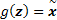.

Although the latent vector has low dimension, it has sufficient size to allow the decoder to recover the input data.

The goal of the decoder is to make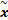as close as possible to x. Generally, both encoder and decoder are non-linear functions. The dimension of z is a measure of the number of salient features it can represent. The dimension is usually much smaller than the input dimensions for efficiency and in order to constrain the latent code to learn only the most salient properties of the input distribution. The autoencoder has the tendency to memorize the input when the dimension of the latent code is much bigger than x.

A suitable loss function,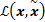, is a measure of how dissimilar the input, x, from the output which is the recovered input,. As shown in Equation 1.1, Mean Squared Error (MSE) is an example of such a loss function:Where m is the output dimensions (for example, in MNIST
m = width × height × channels = 28 × 28 × 1 = 784).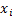and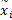are the elements of x andrespectively. Since the loss function is a measure of dissimilarity between input and output, we can use alternative reconstruction loss functions such as binary cross entropy or structural similarity index (SSIM)

Similar to other neural networks, the autoencoder tries to make this error or loss function as small as possible during training. Figure 1 shows the autoencoder. The encoder is a function that compresses the input, x, into a low-dimensional latent vector, z. The latent vector represents the important features of the input distribution. The decoder tries to recover the original input from the latent vector in the form ofFigure 1: Block diagram of an autoencoderFigure 2: An autoencoder with MNIST digit input and output.
The latent vector is 16-dim.

To put the autoencoder in context, x can be a MNIST digit which has a dimension of 28 × 28 × 1 = 784. The encoder transforms the input into a low-dimensional z that can be a 16-dimension latent vector. The decoder attempts to recover the input in the form offrom z. Visually, every MNIST digit x appear similar to. Figure 2 demonstrates the autoencoding process. We can observe that the decoded digit 7 is not exactly the same but close enough.

Since both encoder and decoder are non-linear functions, we can use neural networks to implement both. For example, in the MNIST dataset, the autoencoder can be implemented by MLP or CNN. The autoencoder can be trained by minimizing the loss function through backpropagation. Similar to other neural networks, the only requirement is that the loss function must be differentiable.

If we treat the input as a distribution, we can interpret the encoder as an encoder of distribution,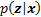and the decoder as the decoder of distribution,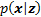. The loss function of the autoencoder is expressed as: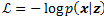The loss function simply means that we would like to maximize the chances of recovering the input distribution given the latent vector distribution. If the decoder output distribution is assumed to be Gaussian, the loss function boils down to MSE since:where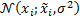is a Gaussian distribution with mean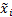and variance of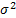. A constant variance is assumed. The decoder outputis assumed to be id. m is the output dimension.

Last Updated ( Sunday, 19 May 2019 )